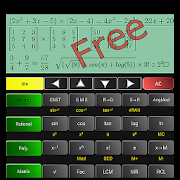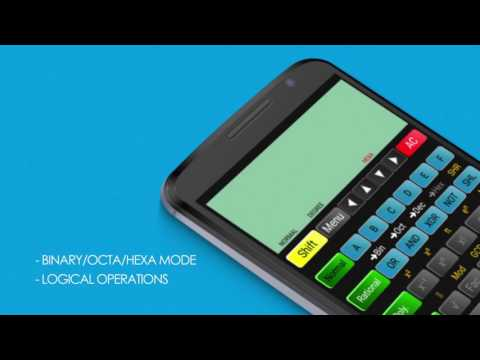# Super Scientific CalculatorEveryone
415Super Scientific calculator is the best free scientific calc with different modes, including:
- Natural mathematical expressions calculation 32 digit with excellent precision
- Polynomial including some algebraic calculation like quadratic equations, evaluation of a polynomial expression and their arithmetic operations, next release will get differentiation of expression as well.
- Rational mode which includes simplification of rational (fractional expressions) so you can simplify a fraction to decimal, and compare two fractions along with their arithmetic operations.
- Matrix mode. This includes matrix arithmetic, inverse of matrix, and adjutant of matrix, determinant of matrix, QR decomposition and Transpose.

Display is quite natural along with look and feel of the layout please see screenshots and video.
Salient features include:
1. Writing and evaluation of whole expressions.
2. Use of brackets.
3. LCM (Least Common Multiple), GCD (greatest common divisor).
4. Binary, Octa and Hexa modes with different bitwise operators of octal, binary and hexadecimal numbers, convert base numbers to decimal and vice versa.
5. factors of a natural number.
6. Trigonometric functions, inverse trigonometry function.
8. Memory functions along with history for up to last 100 calculations.
9. nCr, nPr calculation.
10. Polar to Rectangular and Rectangular to Polar conversion
11. Powers, roots and logarithms including natural logarithms as well.
12. Result history for natural expressions including Navigations.
13. List of different physical constants.
14. Conversion of fractions into decimal numbers.
15. Comparison between two fractions (Rational numbers).
16. Arithmetic of two polynomial expressions
18. Evaluation of any polynomial expression by substituting the value of unknown x.
And many more.

If you feel Super scientific calculator as a great app developed by NTSoft Apps, please rate this app and do provide some suggestions for future improvement, suggestions and bugs report to fix them as soon as possible.
Collapse

Review Policy
4.4
415 total
5
4
3
2
1

## What's New

Adding a complete STATS mode, which includes many statistical functions.
Collapse

Updated
August 19, 2018
Size
6.1M
Installs
50,000+
Current Version
2.2
Requires Android
4.4 and up
Content Rating
Everyone
Permissions
Offered By
NTsoft apps